# 活性炭/壳聚糖多孔小球作为去除阳离子染料的高效吸附剂：等温线，动力学和热力学研究Activated Carbon/Chitosan Porous Beads as a High Efficiency Adsorbent for Cationic Dye Removal: Isotherm, Kinetic, and Thermodynamic Studies

DOI: 10.12677/MS.2018.87097, PDF, HTML, XML, 下载: 633  浏览: 1,070  国家自然科学基金支持

Abstract: The activated carbon/chitosan (AC/CHI) composite porous beads were prepared by cross-linking, curing and freeze-drying methods and were used as an effective adsorbent for the removal of methylene blue (MB) from aqueous solution. The properties of the synthesized AC/CHI were characterized by scanning electron microscope (SEM) and Fourier transform infrared spectroscopy (FTIR). The effect factors including temperature, dosage, contact time, and pH on the adsorption properties of methylene blue onto AC/CHI were studied. The adsorption equilibrium data of methylene blue onto activated carbon were well fitted both to the Langmuir model and Freundlich model, giving a maximum adsorption capacity of 1194.33 mg/g. It indicates that AC/CHI is a promising adsorbent for removing methylene blue from aqueous solution. The kinetic data were well described by the pseudo-second-order model. Thermodynamic parameters indicate that ad-sorption process is spontaneous and endothermic.

1. 引言

2. 实验

2.1. 主要原料与仪器

2.2. 壳聚糖溶液的制备

2.3. 活性炭/壳聚糖多孔小球的制备

2.4. 吸附剂的表征

2.5. 吸附实验

${q}_{e}=\left(\frac{{C}_{0}-{C}_{e}}{W}\right)×V$ (1)

${q}_{t}=\left(\frac{{C}_{0}-{C}_{t}}{W}\right)×V$ (2)

3. 结果与讨论

3.1. 吸附剂的表征

3.2. 不同活性炭含量对吸附性能的影响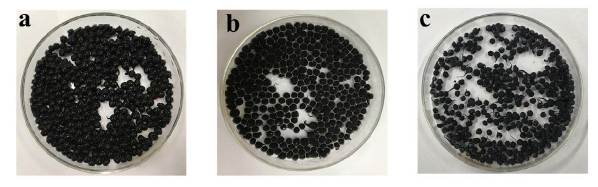Figure 1. Photographs of AC/CHI (a) The curing; (b) The frozen in a refrigerator; (c) The freeze dried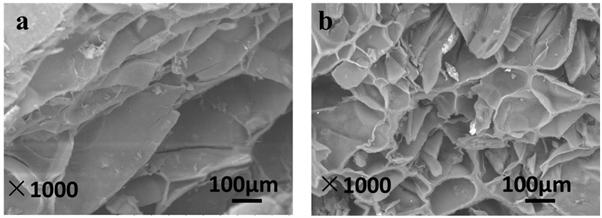Figure 2. SEM images of (a) CHI; and (b) AC/CHI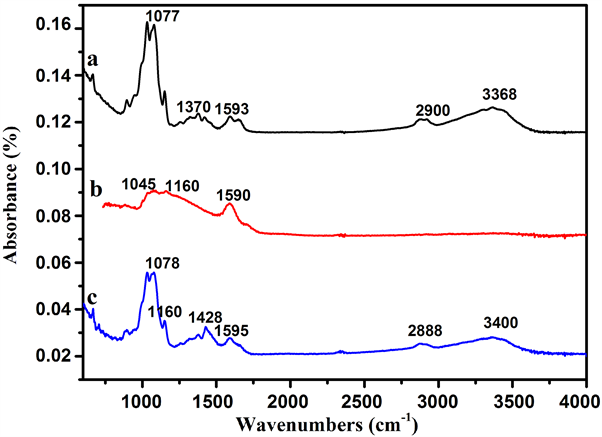Figure 3. FT-IR spectra of (a) CHI; (b) AC; and (c) AC/CHI

3.3. 温度对吸附性能的影响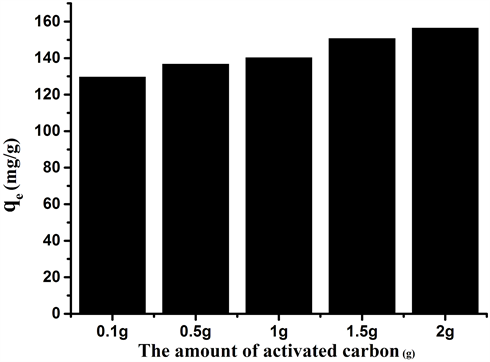Figure 4. Adsorption of methylene blue with different AC content (initial methylene blue concentration: 160 mg/L, adsorption dose: 10 mg, temperature: 303 K, pH: 6.5)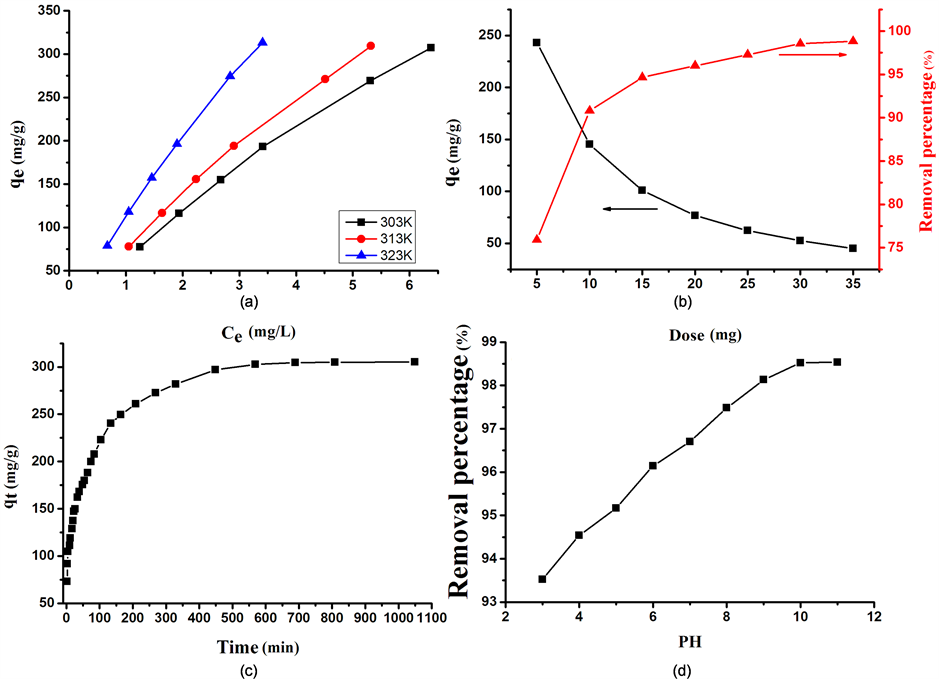Figure 5. Effect of different factors on MB adsorbed by AC/CHI: (a) Temperature effect; (b) Dose effect; (c) Contact time effect; (d) pH effect

3.4. 吸附剂加入量对吸附性能的影响

3.5. 接触时间对吸附性能的影响

3.6. 溶液的初始pH值对吸附的影响

3.7. 吸附等温线研究

$\frac{{C}_{e}}{{q}_{e}}=\frac{{C}_{e}}{{q}_{\mathrm{max}}}+\frac{1}{{q}_{\mathrm{max}}{k}_{L}}$ (3)

${R}_{L}=\frac{1}{1+{k}_{L}{C}_{0}}$ (4)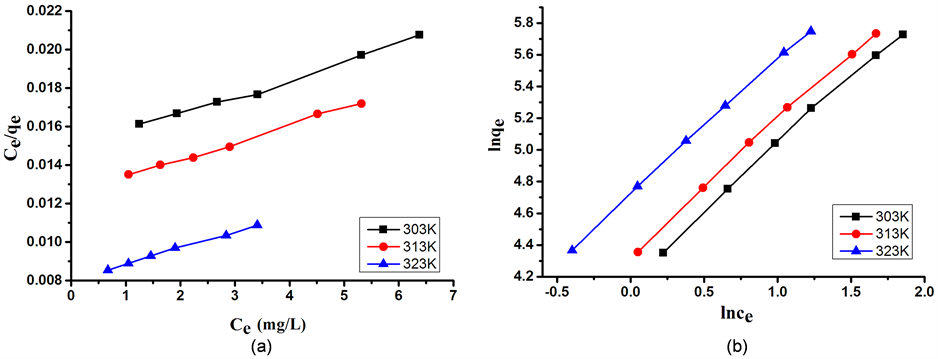Figure 6. The equilibrium plots for the adsorption MB on to the AC/CHI: (a) Langmuir model equilibrium; (b) Freundlich model equilibrium plotsTable 1. The adsorption isotherm constants of Langmuir and Freundlich model for MB onto AC/CHI

$\mathrm{ln}{q}_{e}=\mathrm{ln}{k}_{F}+\frac{1}{n}\mathrm{ln}{C}_{e}$ (5)

3.8. 吸附动力学研究

$\mathrm{log}\left({q}_{e}-{q}_{t}\right)=\mathrm{log}{q}_{e}-\frac{{k}_{1}}{2.303}t$ (6)

$\frac{t}{{q}_{t}}=\frac{1}{{k}_{2}{q}_{e}{}^{2}}+\frac{t}{{q}_{e}}$ (7)

${q}_{t}={k}_{id}{t}^{1}{2}}+C$ (8)

3.9. 吸附热力学研究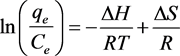(9)

$\Delta G=\Delta H-T\Delta S$ (10)

4. 结论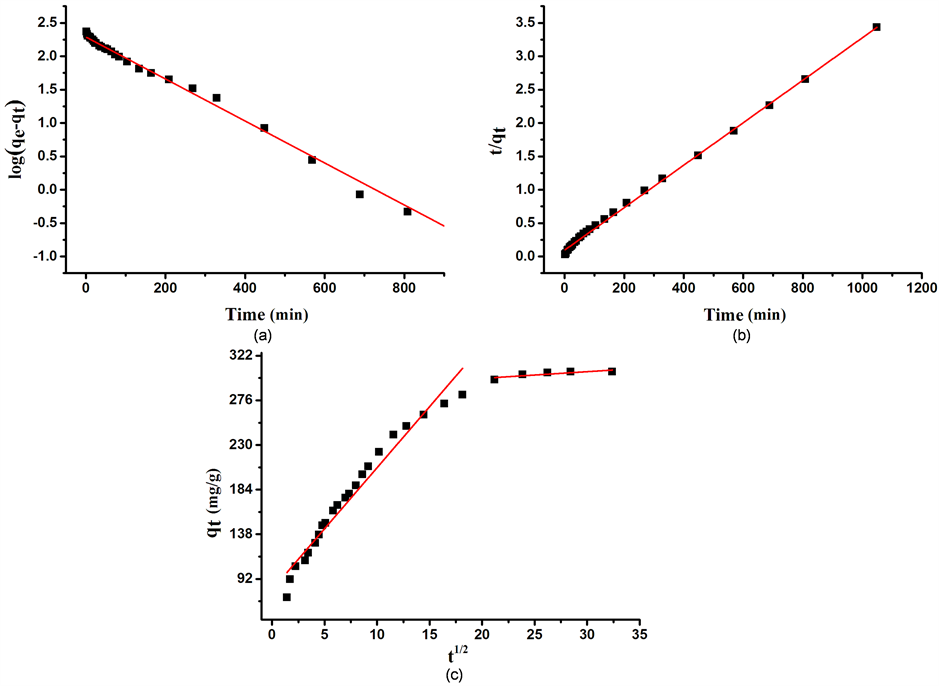Figure 7. Adsorption kinetics of MB adsorbed by AC/CHI: (a) Pseudo-first-order model; (b) Pseudo-second-order model; (c) Intra-particle diffusion modelTable 2. The parameters of pseudo-first-order, pseudo-second-order and intra-particle diffusion modelsTable 3. Thermodynamic parameters at different temperatures

NOTES

*通讯作者。